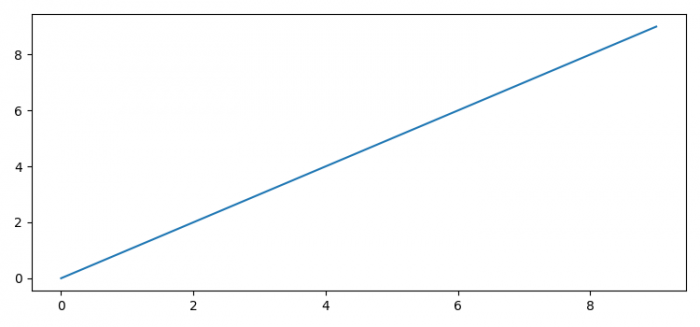# Store mouse click event coordinates with Matplotlib

To store mouse event coordinates with matplotlib, we can use "button_press_event" event.−

## Steps

• Set the figure size and adjust the padding between and around the subplots.
• Create a figure and a set of subplots.
• Plot a line in the range of 10
• Bind the function *onclick* to the event *button_press_event*.
• Print the x and y data of the event.
• To display the figure, use show() method.

## Example

from matplotlib import pyplot as plt

plt.rcParams['backend'] = 'TkAgg'
plt.rcParams["figure.figsize"] = [7.50, 3.50]
plt.rcParams["figure.autolayout"] = True

# Function to print mouse click event coordinates
def onclick(event):
print([event.xdata, event.ydata])

# Create a figure and a set of subplots
fig, ax = plt.subplots()

# Plot a line in the range of 10
ax.plot(range(10))

# Bind the button_press_event with the onclick() method
fig.canvas.mpl_connect('button_press_event', onclick)

# Display the plot
plt.show()

## Output

On execution, it will produce the following output:Now, click anywhere on the plot and it will display that particular point's coordinates on the console:

[6.277811659536052 6.218189947945731]
[4.9416949672083685 3.7079096112932475]
[8.221254287227506 3.4145010811941963]